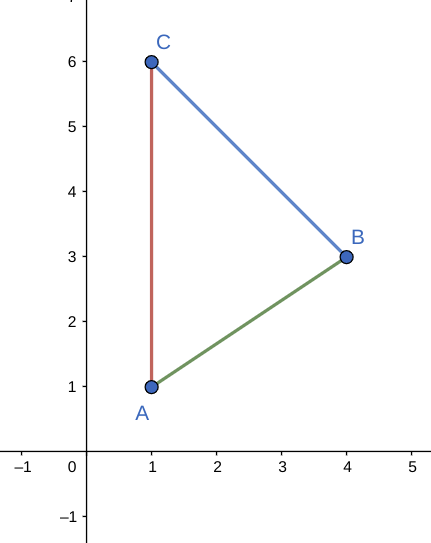# The parametric equations x = x_{1} + \langle x_{2} - x_{1} \rangle t, y = y_{1} + \langle y_{2}...

## Question:

The parametric equations

$$x = x_{1} + \langle x_{2} - x_{1} \rangle t, \, y = y_{1} + \langle y_{2} - y_{1} \rangle t$$

where {eq}0 \leq t \leq 1 {/eq} describe the line segment that joins the points {eq}P_{1}(x_{1}, y_{1}) {/eq} and {eq}P_{2}(x_{2}, y_{2}) {/eq}.

Draw the triangle with vertices {eq}A(1, 1), \, B(4, 3), \, C(1, 6) {/eq}. Find the parametrization, including endpoints, and sketch to check. (Let {eq}x {/eq} and {eq}y {/eq} be in terms of {eq}t {/eq}.)

## Parametric Curves of Line Segments

To find a parametrization of a line segment between two given points we will look for linear functions of a single parameter, t

that describe the line segment, and set up the interval for t such that the start and end point of the parametrization coincide with the coordinates of the two given points.

A parametric vector equation of the line segment from {eq}\displaystyle A =(1,1) \text{ to } B=(4,3) , {/eq} is given by

{eq}\displaystyle \mathbf{r}(t) =\langle 1+(4-1)t, 1+(3-1)t\rangle=\boxed{\langle 1+3t, 1+2t\rangle, 0\leq t\leq 1}. {/eq}

and a parametric equation of the line segment through {eq}\displaystyle B=(4,3) \text{ and }C=(1,6 ) {/eq} is given as

{eq}\displaystyle \mathbf{r}(t) =\langle 4+(1-4)t, 3+(6-3)t\rangle=\boxed{\langle 4-3t, 3+3t\rangle, 0\leq t\leq 1}. {/eq}

A parametrization for the line segment through {eq}\displaystyle C=(1,6 ) \text{ and } A(1,1) {/eq} is given as

{eq}\displaystyle \mathbf{r}(t) =\langle 1+(1-1)t, 6+(1-6)t\rangle=\boxed{\langle 1, 6-5t\rangle, 0\leq t\leq 1}. {/eq}

The three segments of the triangle are shown in the figure below,